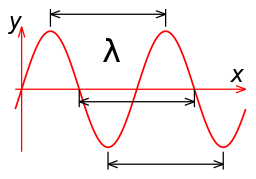# Frequency to Wavelength Calculator

This tool converts Frequency to Wavelength.

## Formula

Wavelength is given by the formula

λ = c/f

where, c is the speed of light and f is the frequency. In a vacuum, the speed of light is c = 299,792,458 meters/second. It is slower in other media such as in glass for instance where the speed is 200,000,000 meters/second. Change the value of c in the calculator above to calculate the frequency in this case.

The picture below shows the wavelength (λ) of a sine waveDicklyon (Richard F. Lyon), CC BY-SA 3.0, via Wikimedia Commons

## Frequency and Wavelength in RF Engineering

Most Radio engineers are used to referring to regions of the electromagnetic spectrum in terms frequency – for instance the Wi-Fi band is 2.4 GHz and 5 GHz. The exception is when you get to lower frequencies in HF and VHF. In those situations, engineers, hobbyists and technologists refer to the wavelength. For instance the 2 meter and 70 cm bands. It can be a little confusing sometimes (it was for me at least). I use this calculator to clear that up.